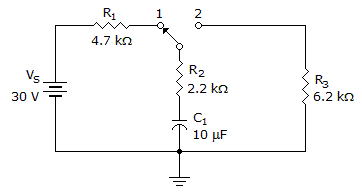# Electronics - Capacitors

### Exercise :: Capacitors - General Questions

46.How long would it take the capacitor in the given circuit to completely charge if the switch was in position 1?

 A. 22 ms B. 69 ms C. 345 ms D. 440 ms

Explanation:

No answer description available for this question. Let us discuss.

47.

An 8 V power source is charging a capacitor. How many volts will be across the capacitor at the second time constant?

 A. 1 volt B. 3 volts C. 5 volts D. 7 volts

Explanation:

No answer description available for this question. Let us discuss.

48.

The material used between the plates of a capacitor is called its:

 A. insulation material B. dielectric material C. separation material D. plate-divider material

Explanation:

No answer description available for this question. Let us discuss.

49.

When a circuit consists of a capacitor and a resistor in series with a voltage source, and the voltage across the resistor is zero, then the capacitor is:

 A. charging B. discharging C. fully charged D. fully discharged

Explanation:

No answer description available for this question. Let us discuss.

50.

Which material has the highest dielectric strength?

 A. Air B. Paper C. Mica D. Oil